• VEC = PLANENORMVEC(PT1,PT2,PT3) 计算包含三个点 PT1、PT2 和 PT3 的平面的法向量。 当平面表示为 ax + by + cz = 1 时，VEC(1) = a，VEC(2) = b，VEC(3) = c。 点应该是一个 1 x 3 的向量，为每一列指定 x、y 和 z...matlab
• THREEPOINTS2PLANEZ返回通过3D空间中三个点平面方程的系数。 平面方程的形式为 z =斜率x * x +斜率* y + C 例子： 给定 x 和 y 中的三个随机点，定义在 x 方向具有 2.1 斜率和在 y 方向具有 -3.4 斜率的 z 平面。...matlab
• matlab 上三点画圆弧的程序，输入三个点坐标即可。
• 如何在vtk中根据三点提取一个切面
如何在vtk中根据三点提取一个切面，一直知道根据三点就能表示一个面，但是怎么在vtk中实现，对于我这种菜鸟真是要想好久。首先说说现在的思路，知道vtkImagepalne中可以根据一个点和一个面法向量获得一个面。现在我有三个点，需要获得一个面法向量，那么需要通过叉乘得到，再然后根据一个点和得到的面法向量显示一个切面。
之前看过vtkresliceImage得到的切面方法，感觉对变换的理解很差，尤其对Matrix（矩阵基本变换）的理解又不那么到位：  double center;  center = origin + spacing * 0.5 * (extent + extent);  center = origin + spacing * 0.5 * (extent + extent);  center = origin + spacing * 0.5 * (extent + extent)；  static double axialElements = {  1, 0, 0, 0,  0, 1, 0, 0,  0, 0, 1, 0,  0, 0, 0, 1 };  vtkSmartPointer<vtkMatrix4x4> resliceAxes =  vtkSmartPointer<vtkMatrix4x4>::New();  resliceAxes->DeepCopy(axialElements);  resliceAxes->SetElement(0, 3, center);  resliceAxes->SetElement(1, 3, center);  resliceAxes->SetElement(2, 3, center);  根据代码的理解，vtkMatrix4x4应该包含一个面和一个点，但是这个面是由static double axialElements = {  1, 0, 0, 0,  0, 1, 0, 0,  0, 0, 1, 0,  0, 0, 0, 1 };    决定的，根据定义，这个矩阵左上方3x3的矩阵是X、Y、Z方向的三个矢量，第四列三个做透视变换用的，根据水灵书中提的，这里好像是切面的坐标系原点（我没有看过透视变换的相关信息，不太了解）。第四行左三个别控制其在x轴y轴z轴上的平移单位.，第四个表示缩放比例。
再理解一下叉乘，叉乘（cross product）或者外积，它的计算结果是一个向量而非标量。叉积所在的向量与参与运算的两个向量都正交，也即正交于原来的两个向两边所决定的平面，也即两向量所决定的平面的法向量可通过计算叉积的方式得以确定。当参与运算的两向量是平行的两个向量时，得到的叉积为0，也即可通过计算叉积的方式判断两向量是否平行。  代数定义  二维时  x×y=x1y2−x2y1  三维时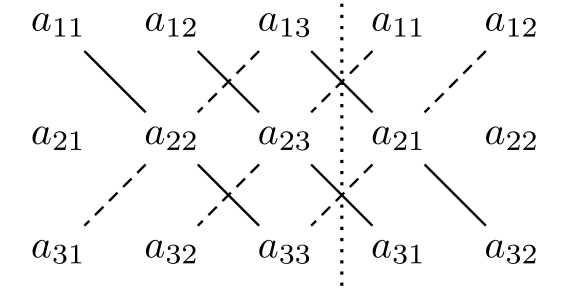这里写图片描述  根据如图的计算方法可得：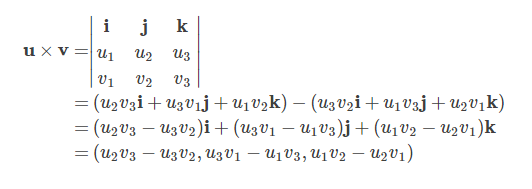几何定义
x×y=∥x∥∥y∥sinθn⃗  n⃗ 表示叉积方向上的单位向量。
中学知识告诉我们三角形的面积计算公式为：
S=∥x∥∥y∥sinθ2=∥z∥h2
其中θ表示的是x,y之间的夹角，由以上两个公式我们可得到三角形的高或者点到其所对的边的距离，也即点到直线的距离，的计算公式：  h=∥x×y∥/∥z∥
double operator*(const Vec& x, const Vec& y)
{
assert(x.size() == y.size());       // #include <cassert>
double sum = 0.;
for (size_t i = 0; i < x.size(); ++i)
sum += x[i]*y[i];
return sum;
}

// 三维的情况
Vec operator^(const Vec& x, const Vec& y)
{
assert(x.size() == y.size() && x.size() == 3);
return Vec{x*y-x*y,
x*y-x*y,
x*y-x*y};
// uniform initialization, C++11新特性
}

// 二维就姑且返回其模长吧
double twoDCrossProd(const Vec& x, const Vec& y)
{
return x*y-x*y;
}
现在贴一段代码[C++实现叉乘]，并感谢作者提供。(http://blog.sina.com.cn/s/blog_4e24d9c50100ttvz.html)
#include <iostream>
#define M 3
#define N 4
#define P 3
using namespace std;
void getmt(int* m,int r,int c)              //获取矩阵
{
for(int i=0;i<r;++i)
{
for(int j=0;j<c;++j) cin>>*(m+i*c+j);
}
}
void showmt(const int* m,int r,int c)      //显示矩阵
{
for(int i=0;i<r;++i)
{
for(int j=0;j<c;++j) cout<<*(m+i*c+j)<<"\t";
cout<<endl;
}
}
void mmt(const int* m1,const int* m2,int* m3,int m,int n,int p)
{
for(int i=0;i<m;++i)                 //叉乘运算
{
for(int j=0;j<p;++j)
{
for(int k=0;k<n;++k)
{
(*(m3+i*p+j))+=(*(m1+i*n+k))*(*(m2+k*p+j));
}
}
}
}
int main(int argc, char *argv[])
{
int m1[M][N];
int m2[N][P];
int m3[M][P];
memset(m3, 0, sizeof(m3));    //注意不能用int m3[M][P]={0};来初始化为0,这样可能并没有将m3中的元素赋0
cout<<"为矩阵1输入"<<M<<"*"<<N<<"个数："<<endl;
getmt(&m1,M,N);        //获取矩阵m1
cout<<"为矩阵2输入"<<N<<"*"<<P<<"个数："<<endl;
getmt(&m2,N,P);        //获取矩阵m2
mmt(&m1,&m2,&m3,M,N,P);   //叉乘运算
cout<<"矩阵1："<<endl;
showmt(&m1,M,N);
cout<<"矩阵2："<<endl;
showmt(&m2,N,P);
cout<<"结果："<<endl;
showmt(&m3,M,P);
system("PAUSE");
return EXIT_SUCCESS;
}
搜了一下在图形学中表示向量的方法，看来这里面学问挺多的，贴两个实用的帖子，感谢作者提供宝贵的知识图形学中向量操作类、图像学中向量说明。
vtkImagePlaneWidget中不能直接设置SetNormal和SetCenter，只能设置初始点位置，那么这样就需要写一个类来继承vtkImagePlaneWidget，并且添加这两个功能。最后，继承了vtkPlaneSource中SetNormal和SetCenter这两个功能，可以提取经过三个点的切面：
    ImagePlaneWidget *mplanewidget = ImagePlaneWidget::New();
mplanewidget->SetInteractor(iren);
mplanewidget->SetPicker(picker); //定义 内部选择器
mplanewidget->SetTexturePlaneProperty(ipwProp);//给切片设定纹理属性
mplanewidget->TextureInterpolateOff();
mplanewidget->GetPlaneProperty()->SetColor(1, 0, 0); //设置平面属性
mplanewidget->SetResliceInterpolateToLinear();//差值方法
//mplanewidget->RestrictPlaneToVolumeOn(); //确保平面在容器范围之内
mplanewidget->SetNormal(mnormalize);  //设定切面法向方向
mplanewidget->SetOrigin(moriginpoint); //设定切面的初始点位置
mplanewidget->SetCenter(moriginpoint);  //设定切面所在位置中心
mplanewidget->On();
mplanewidget->InteractionOn();
//mplanewidget->PlaceWidget(polyData->GetBounds());//获得polydata中的边界，最大最小值
展开全文vtk
• 　众所周知，在维空间内，不共线的三个点可以确定一个平面，现在给出三个点的空间坐标[x1,y1,z1]，[x2,y2,z2]，[x3,y3,z3]，请计算在这3个点确定平面上坐标分量x, y, z分别在[lx, rx]，[ly, ry]，[lz,rz]内的...Golang erlang r语言
• ## 三个点确定一个圆

千次阅读 2017-10-01 08:17:06
首先假设圆心为(x0, y0)，半径为r。则这个圆可以表示为： ...假设知道的三个点为(x1, y1)，(x2,y2)，(x3,y3)。那么： (x1−x0)2+(y1−y0)2=r2(x1 - x0)^2+(y1 - y0)^2 = r^2 (x2−x0)2+(y2−y0)2=r2(x2 - x0)^2+
首先假设圆心为(x0, y0)，半径为r。则这个圆可以表示为：

(x−x0)2+(y−y0)2=r2​
$(x - x0)^2+(y - y0)^2 = r^2​$
假设知道的三个点为(x1, y1)，(x2,y2)，(x3,y3)。那么：

(x1−x0)2+(y1−y0)2=r2
$(x1 - x0)^2+(y1 - y0)^2 = r^2$

(x2−x0)2+(y2−y0)2=r2
$(x2 - x0)^2+(y2 - y0)^2 = r^2$

(x3−x0)2+(y3−y0)2=r2
$(x3 - x0)^2+(y3 - y0)^2 = r^2$
消去

r2
$r^2$后变成

(x1−x0)2+(y1−y0)2=(x2−x0)2+(y2−y0)2
$(x1 - x0)^2+(y1 - y0)^2 = (x2 - x0)^2+(y2 - y0)^2$

(x1−x0)2+(y1−y0)2=(x3−x0)2+(y3−y0)2
$(x1 - x0)^2+(y1 - y0)^2 = (x3 - x0)^2+(y3 - y0)^2$
将未知量x0,y0移到左边就变为

(x1−x2)x0+(y1−y2)y0=(x12−x22)+(y12−y22)2
$(x1 - x2)x0 + (y1 - y2)y0 = \frac{(x1^2 - x2^2) + (y1^2 - y2^2)}{2}$

(x1−x3)x0+(y1−y3)y0=(x12−x32)+(y12−y32)2
$(x1 - x3)x0 + (y1 - y3)y0 = \frac{(x1^2 - x3^2) + (y1^2 - y3^2)}{2}$
首先我们要确定这个方程是不是有解，很简单，即判断行列式

∣∣∣(x1−x2)(x1−x3)(y1−y2)(y1−y3)∣∣∣≠0
$\left| \begin{array} ((x1 - x2) & (y1 - y2) \\(x1 - x3) & (y1 - y3) \end{array} \right | \neq 0$
设两个常量a1,a2

a1=(x12−x22)+(y12−y22)2
$a1 = \frac{(x1^2 - x2^2) + (y1^2 - y2^2)}{2}$

a2=(x12−x32)+(y12−y32)2
$a2 = \frac{(x1^2 - x3^2) + (y1^2 - y3^2)}{2}$
那么

x0=(y1−y2)a2−(y1−y3)a1(y1−y2)(x1−x3)−(x1−x2)(y1−y3)
$x0 = \frac{(y1 - y2)a2 - (y1 - y3)a1}{(y1 - y2)(x1 - x3)-(x1 - x2)(y1-y3)}$

y0=(x1−x3)a1−(x1−x2)a2(y1−y2)(x1−x3)−(x1−x2)(y1−y3)
$y0 = \frac{(x1 - x3)a1 - (x1 - x2)a2}{(y1 - y2)(x1 - x3)-(x1 - x2)(y1-y3)}$
最后代码如下
import numpy as np
import matplotlib.pyplot as plt
from matplotlib.patches import Circle
#www.coordinate.wang
def circle(x1, y1, x2, y2, x3, y3):
a = x1 - x2
b = y1 - y2
c = x1 - x3
d = y1 - y3
a1 = ((x1 * x1 - x2 * x2) + (y1 * y1 - y2 * y2)) / 2.0
a2 = ((x1 * x1 - x3 * x3) + (y1 * y1 - y3 * y3)) / 2.0
theta = b * c - a * d;
if abs(theta) < 1e-7:
return -1
x0 = (b * a2 - d * a1) / theta;
y0 = (c * a1 - a * a2) / theta;
r = np.sqrt(pow((x1 - x0), 2)+pow((y1 - y0), 2))
fig = plt.figure()
cir = Circle(xy = (x0, y0), radius = r, alpha=0.4)
plt.axis('scaled')
plt.axis('equal')
plt.plot(x1,y1,'r*',x2,y2,'r*',x3,y3,'r*')
plt.show()

circle(0, 0, 0, 1, 1, 0)
结果为展开全文python 算法
• 已知三个点坐标为P1(x1,y1,z1), P2(x2,y2,z2), P3(x3,y3,z3) 所以可以设方程为A(x - x1) + B(y - y1) + C(z - z1) = 0 (点法式) (也可设为过另外两个点) 核心代码： //在此之前写好录入三个三维点...
转载：https://blog.csdn.net/PengPengBlog/article/details/52774421
已知三个点坐标为P1(x1,y1,z1), P2(x2,y2,z2), P3(x3,y3,z3)
所以可以设方程为A(x - x1) + B(y - y1) + C(z - z1) = 0 (点法式) (也可设为过另外两个点)

核心代码：
//在此之前写好录入三个三维点的代码，然后就是处理待定系数，如下：
A = (y2 - y1)*(z3 - z1) - (z2 -z1)*(y3 - y1);
B = (x3 - x1)*(z2 - z1) - (x2 - x1)*(z3 - z1);
C = (x2 - x1)*(y3 - y1) - (x3 - x1)*(y2 - y1);

即得过P1，P2，P3的平面方程
方程也可写为    Ax + By + Cz + D = 0 (一般式)    其中D = -(A * x1 + B * y1 + C * z1)
展开全文• 生成两个点，这个算子在Halcon维中常用。 讲点连接成线 dev_set_line_width(2) *画点 dev_set_color('red') gen_cross_contour_xld(Cross1, 90, 103, 16, 0.785398) gen_cross_contour_xld(Cross2, 377, 341...
一.二维平面确定法向向量
生成两个点，这个算子在Halcon三维中常用。讲点连接成线
    dev_set_line_width(2)
*画点
dev_set_color('red')
gen_cross_contour_xld(Cross1, 90, 103, 16, 0.785398)
gen_cross_contour_xld(Cross2, 377, 341, 16, 0.785398)
gen_contour_polygon_xld (line1, [90,377], [103,341])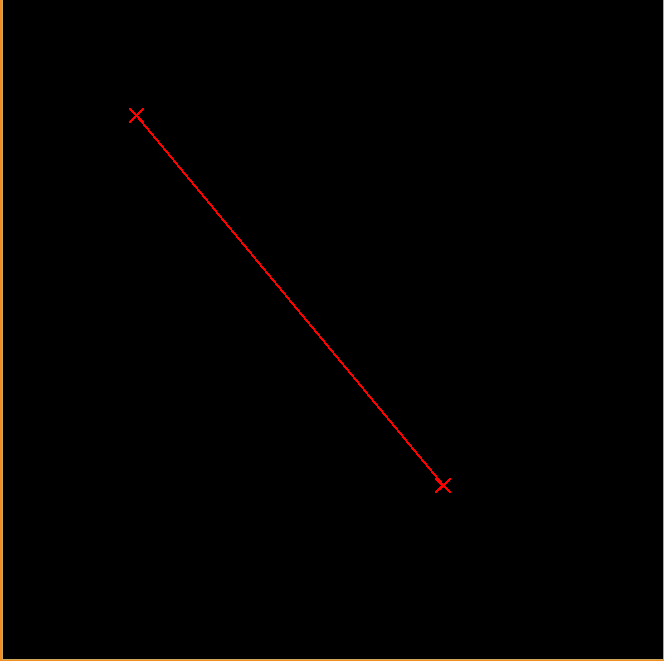生成射线
    dev_set_color('green')
gen_arrow_contour_xld(Arrow, 90, 103, 377, 341, 5, 5)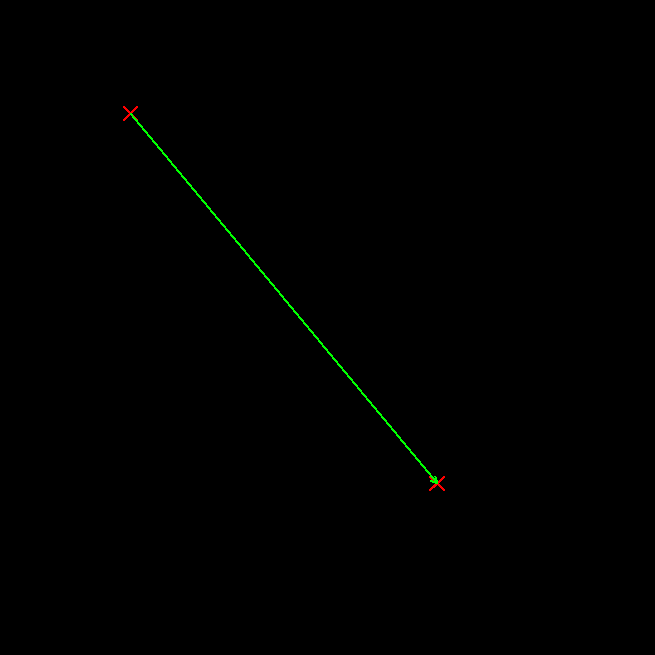生成齐次矩阵，代表两点的方向向量使用矩阵平移点
   vector_angle_to_rigid(90, 103,0 ,377, 341, 0, HomMat2D)
*121 53  这个点
gen_cross_contour_xld(Cross3, 121, 53, 16, 0.785398)
*变换
affine_trans_pixel(HomMat2D, 121, 53, RowTran, ColTran)
*
gen_cross_contour_xld(Cross4, RowTran, ColTran, 16, 0.785398)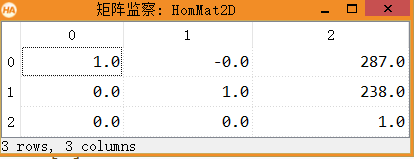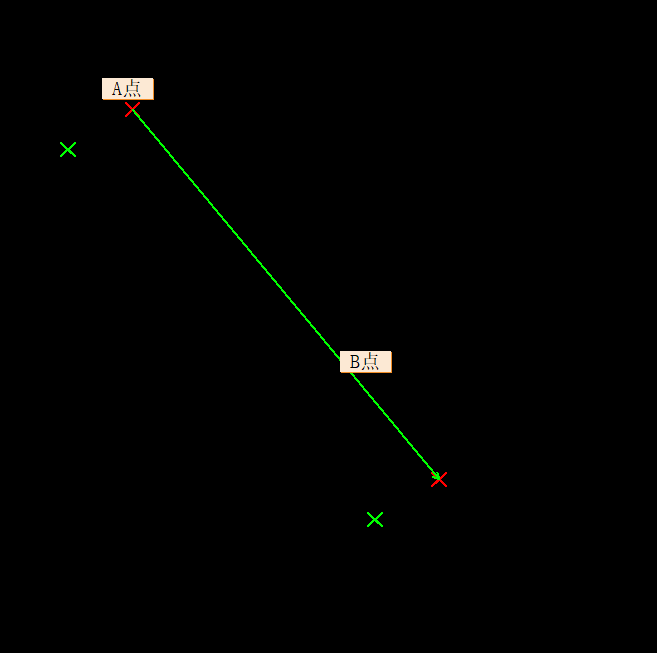二.三维空间确定姿态
绘制空间六个点以及点云外接矩形注意空间点是没有厚度的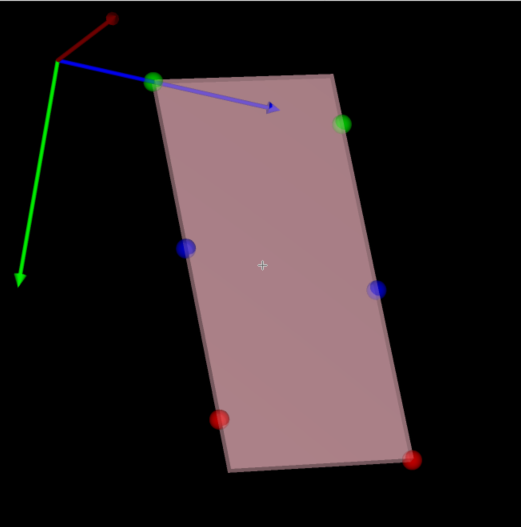通过空间点的Pose，设置平面宽度可以获得一个空间平面。
    poseLeft:= [0,  0, 50,0,90,0,0]
*VisualizationPlaneSize1，VisualizationPlaneSize2分别是平面宽高
gen_plane_object_model_3d (poseLeft, [-1,-1,1,1] * VisualizationPlaneSize1, [-1,1,1,-1] * VisualizationPlaneSize2, IntersectionPlane_Left)


使用第一步获得的外接箱体，将其前三位(x,y,z)，转化为点的位置。
      Pose:=poseMiddle
Pose:=poseMiddle
Pose:=poseMiddle

此时得到的 Pose既可以绘制平面也可以绘制柱体(需要自定义长宽高)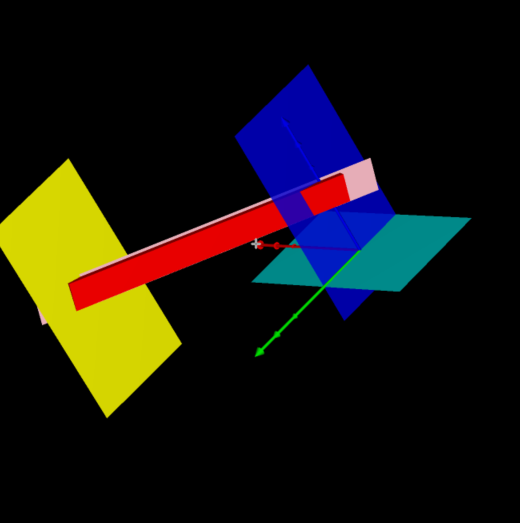掌握这两个技巧是有必要的，需求是鞋底涂胶，机器手的姿态确定
展开全文• Sceneform 框架下通过三个点绘制平面（Plane） 两个点确定一条直线，三个点确定一个平面，绘制直线的比较好处理，凭空添加平面就很少见了，这篇文章就是基于这个需求做的 ...SceneForm
• https://blog.csdn.net/cwj066/article/details/82692271（顺便推荐一个基础计算博客） 这里不讲公式推导数学计算,自己去问别人。博主也不想算，拿来就用。想弄清楚一点的，可以看原理传送门。 C/C++代码实现...
• 给定维空间里的任意三个点确定一个平面方程Ax+By+Cz+D=0的求解过程及伪代码的实现 其思想就是 1.先求解该平面的法向量n 2.由点法式将其中的任意一点代入公式即可以求得平面方程Ax+By+Cz+D=0的系数ABCD 3.伪代码...
• //p1,p2,p3确定平面的法向量 n.x=(p3.y-p2.y)*(p1.z-p2.z)-(p3.z-p2.z)*(p1.y-p2.y); n.y=(p3.z-p2.z)*(p1.x-p2.x)-(p3.x-p2.x)*(p1.z-p2.z); n.z=(p3.x-p2.x)*(p1.y-p2.y)-(p3.y-p2.y)*(p1.x-p2.x); ...
• 已知三个点坐标为P1(x1,y1,z1), P2(x2,y2,z2), P3(x3,y3,z3) 所以可以设方程为A(x - x1) + B(y -...//在此之前写好录入三个三维点的代码，然后就是处理待定系数，如下： A = (y3 - y1)*(z3 - z1) - (z2 -z1)*(y3 - y1);
• 已知三个点的坐标,某未知点与三个已知点的距离,求未知点的坐标。利用三点的其距离，构成圆形的交点，是唯一一点，就是要求的未知点。三点定位
• 已知三点求平面方程、平面法向量和平面...关键在于求出平面一个法向量，为此做向量p1p2（x2-x1,y2-y1,z2-z1),p1p3(x3-x1,y3-y1,z3-z1),平面法线和这两个向量垂直，因此法向量n： 平面方程：a(x-x1)+b(y-y1...
• ②三条直线的斜率都不存在，即三个横坐标相同，三点共线; ③其他情况下：若三条直线的纵坐标相同，三点共线； 否则三点不共线; */ /* 三点是否共线分三种情况考虑： ①当三条直线的斜率都存在时，若三条直线的斜率...算法
• 比如我要在原有的马鞍面上添加一个与X-Z平面平行的平面，例如y=-5这个平面。 下图是origin2017的主界面 在快捷键那一栏中有一个名字为New 3D Plot的下拉菜单，如下图所示，选择New 3D Parametric Plot 选项 接着...
• 已知三点p1,p2,p3,求其平面方程，p1=[x1,y1,z1];p2=[x2,y2,z2];p3=[x3,y3,z3];那么求解下面矩阵q行列式就是了：q=[ones(4,1),[x,y,z];p1;p2;p3];detb=det(q);最后令q=0; 这里的求解方法是四阶矩阵的行列式求解，可以...
• python实现平面的距离 目录 python实现平面的距离 1.三点定面 2.到面的距离 3.python实现到面的距离 ...空间上任意三个不共线的，可以确定一个平面，三点定面的例子： 2.到面的距离 ...
• 平面三点求圆心坐标和半径的C#开发的小程序，可以点击EXE文件直接运行，并带VS源码。
• 需求：给定一个二维平面平面上有 n 个点，求最多有多少个点在同一条直线上。 分析思路： 1、将所有点二维坐标化，即定义出所有点的x，y坐标值 2、遍历出所有取出两点的情况(不考虑先后顺序)，根据任意两点都确定一...
• 思路：1、先求出n个结点中取出三个结点 有多少种方法 C(n,3)的组合； 2、在找出三个点不能组成三角形的情况；有斜率相等和不存在斜率两种情况； 完整代码如下： #include&lt;iostream&gt; #include &...
• 要求确定平面方程,关键在于求出平面一个法向量 为此做向量p1p2（x2-x1,y2-y1,z2-z1), p1p3(x3-x1,y3-y1,z3-z1),平面法线和这两个向量垂直，因此法向量n： 平面方程：a(x-x1)+b(y-y1)+ c(z-z1)=0；...
• 关键在于求出平面一个法向量，为此做向量p1p2（x2-x1,y2-y1,z2-z1), p1p3(x3-x1,y3-y1,z3-z1),平面法线和这两个向量垂直，因此法向量n： //已知3坐标，求平面ax+by+cz+d=0; void get_panel(Point p1c++ 数学
• // fitplane.cpp : 定义控制台应用程序的入口。//#include "stdafx.h"#include&lt;iostream&gt;#include&lt;cmath&gt;typedef struct Point{ double x; double y; double z;};bool ...
• ## 平面三点计算夹角

万次阅读 2017-02-13 21:09:14数学几何 JavaScript
• 最近学图形学时遇到了这个问题，PPT 给的大概是一个通过线性代数的方法求的，有点看不懂。加上线性代数早就忘光了，更加是一脸茫然。但是这个知识在高中讲过，自己却怎么也记不起来了，直到今天突然记起来了，特此...法向量
• ## R中的三个点...

千次阅读 2018-06-25 14:27:30
ff &lt;- function(a, b, c, d){a+b+c+d} ...这里，函数fff()中的参数…和函数ff()中的…表示相同的参数，这里是指b,c,d三个参数，要注意参数的数量和位置需要相对应。当参数较多时，可以这样使用。...r
• 纯哥想知道，平面上的三个点是否在一条直线上 输入 一个正整数n，表示n组案例。 接下来是n行，每行代表一组案例，由6个整数a、b、c、d、e、f构成，代表平面直角坐标系上的三个点(a,b), (c,d), (e,f)。 输出 针对每组......